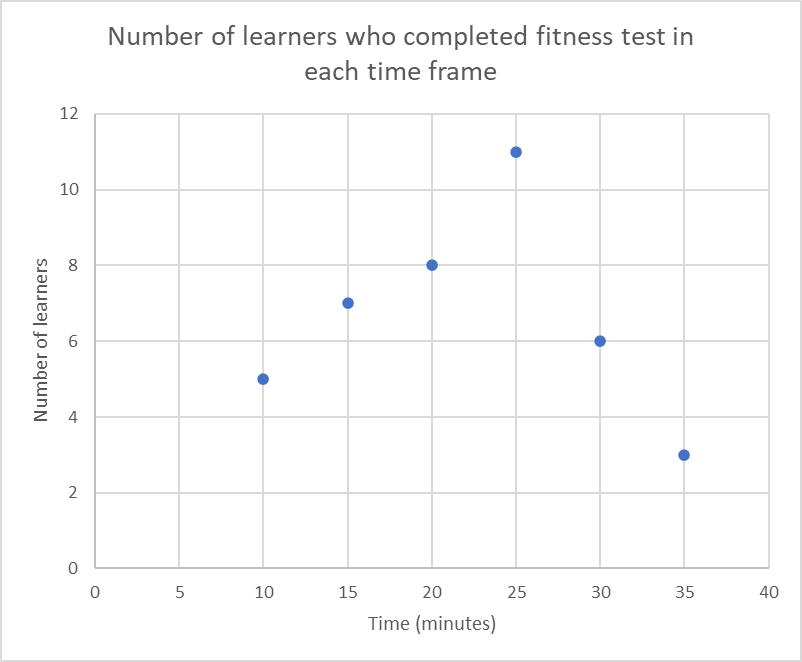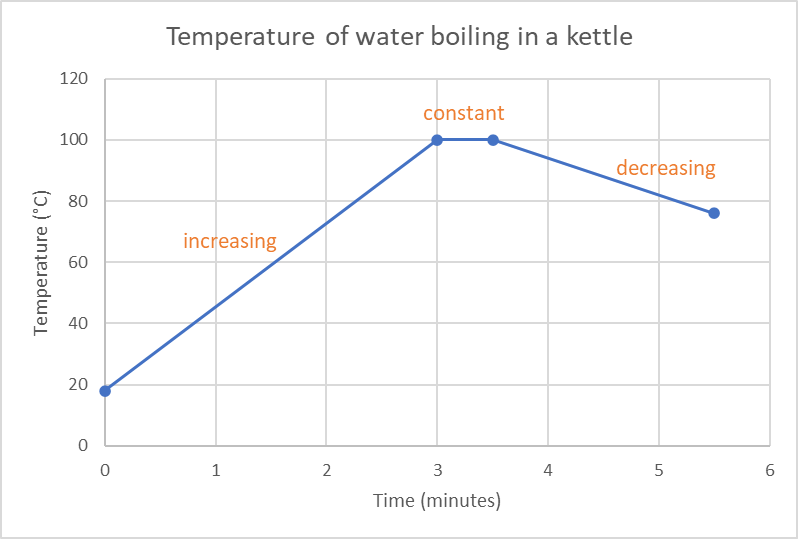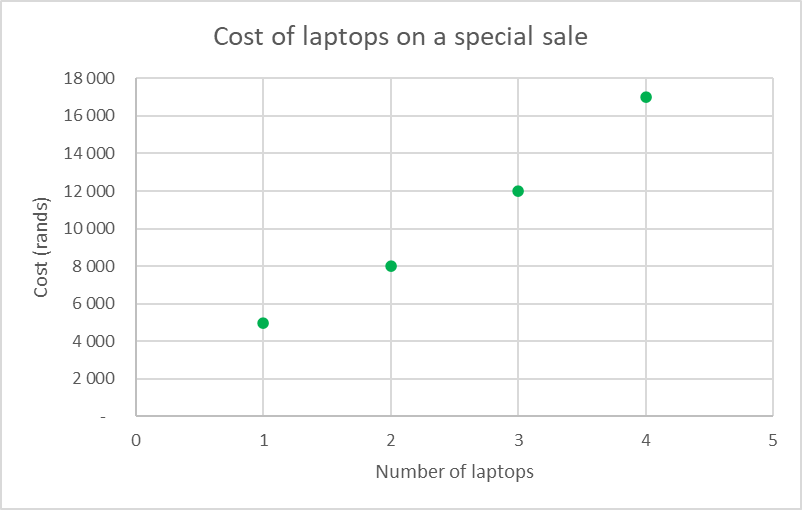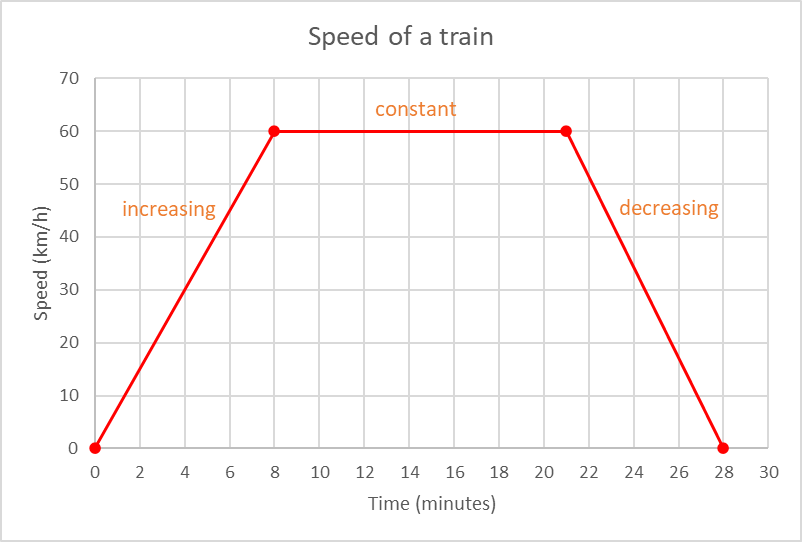Home Practice
For learners and parents For teachers and schools
Textbooks
Full catalogue
Pricing SupportLog in

We think you are located in United States. Is this correct?

# Drawing global graphs

## 23.4 Drawing global graphs

If there is enough information provided in the description of a situation or the result of an event, we can use the data to draw a graph.

## Worked example 23.2: Drawing a graph from a problem statement

Forty learners completed a physical fitness test consisting of different exercises. $$5$$ learners completed the test in $$10$$ minutes, $$7$$ learners completed the test in $$15$$ minutes, $$8$$ learners completed the test in $$20$$ minutes, $$11$$ learners completed the test in $$25$$ minutes, $$6$$ learners completed the test in $$30$$ minutes and $$3$$ learners completed the test in $$35$$ minutes.

Use the information provided to answer the questions.

1. Is the data in this situation discrete or continuous?
2. Use the information provided to draw a suitable graph.
3. How many learners completed the test in $$25$$ minutes or less?

### Identify the type of data.

The data is discrete. Time is the independent variable and the number of learners to complete the test is the dependent variable. The number of learners can be counted.

### Use the data to draw a graph.

The data is discrete so the points are not joined by a line.

### Find the total number of learners who completed the test in 25 minutes or less.From the graph we can add together the number of learners who completed the test in $$25$$ minutes or less: $$5 + 7 + 8 + 11 = 31$$ learners.

Alternatively, we can subtract the number of learners who took longer than $$25$$ minutes to complete the test from the total number of learners:

\begin{align} 40 - (6 + 3) & = 40 - 9 \\ & = 31 \text{ learners} \end{align}

# Test yourself now

High marks in science are the key to your success and future plans. Test yourself and learn more on Siyavula Practice.

Exercise 23.2

The starting temperature of water in a kettle is $$18 ^{ \circ}\text{C}$$. When the kettle is turned on, the temperature increases at a steady rate for 3 minutes until it reaches $$100 ^{ \circ}\text{C}$$. It boils for 30 seconds and then switches off. After 2 minutes, the temperature of the water in the kettle has cooled to $$76^{ \circ}\text{C}$$.

1. Is the data in this situation discrete or continuous?
2. Use the information provided to draw a suitable graph.
3. Indicate on your graph the sections where the graph is increasing, decreasing and constant.
1. Continuous
2. and 3.A computer shop is offering a special deal on laptops: One laptop costs $$\text{R}\,\text{5 000}$$, 2 laptops cost $$\text{R}\,\text{8 000}$$, three laptops cost $$\text{R}\,\text{12 000}$$ and four laptops cost $$\text{R}\,\text{17 000}$$.

Use the information provided to answer the questions.

1. Is the data in this situation discrete or continuous?
2. Use the information provided to draw a suitable graph.
3. Before the sale started, Maggie bought four laptops at $$\text{R}\,\text{6 500}$$ each. How much would she have saved if she bought the laptops during the sale?
1. Discrete
2.3. $$4 \times \text{R}\,\text{6 500} = \text{R}\,\text{26 000}$$
On sale, four laptops cost $$\text{R}\,\text{17 000}$$
$$\text{R}\,\text{26 000} - \text{R}\,\text{17 000} = \text{R}\,\text{9 000}$$

A train leaves the station and increases its speed at a constant rate for 8 minutes until it reaches $$60 \text{ km}{\cdot}\text{h}^{-1}$$. The train travels at a constant speed of $$60 \text{ km}{\cdot}\text{h}^{-1}$$ for 13 minutes and then reduces its speed at a steady rate. It takes the train 7 minutes to come to a complete stop at the next station.

1. Is the data in this situation discrete or continuous?
2. Use the information provided to draw a suitable graph.
3. On your graph, indicate the sections where the graph is increasing, decreasing and constant.
1. Continuous
2. and 3.